In this Pandas group by we are going to learn how to organize Pandas dataframes by groups. More specifically, we are going to learn how to group by one and multiple columns. Furthermore, we are going to learn how calculate some basics summary statistics (e.g., mean, median), convert Pandas groupby to dataframe, calculate the percentage of observations in each group, and many more useful things.

First of all we are going to import pandas as pd, and read a CSV file, using the read_csv method, to a dataframe. In the example below, we use index_col=0 because the first row in the dataset is the index column.

```import pandas as pd

data_url = 'http://vincentarelbundock.github.io/Rdatasets/csv/carData/Salaries.csv'

##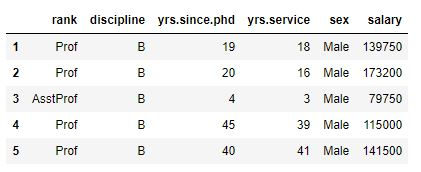We used Pandas head to se the first 5 rows of our dataframe. In the image above we can see that we have, at least, three variables that we can group our data by. That is, we can group our data by “rank”, “discipline”, and “sex”. Of course, we could also group it by yrs.since.phd or yrs.service but it may be a lot of groups.  As previously mentioned we are going to use Pandas groupby to group a dataframe based on one, two, three, or more columns.

Data can be loaded from other file formats as well (e.g., Excel, HTML, JSON):

## Python Pandas Groupby Example

We are starting with the simplest example; grouping by one column. In the Pandas groupby example below we are going to group by the column “rank”.

There are many different methods that we can use on Pandas groupby objects (and Pandas dataframe objects). All available methods on a Python object can be found using this code:

```import IPython

# Grouping by one factor
df_rank = df.groupby('rank')

# Getting all methods from the groupby object:
meth = [method_name for method_name in dir(df_rank)
if callable(getattr(df_rank, method_name)) & ~method_name.startswith('_')]

# Printing the result
print(IPython.utils.text.columnize(meth))```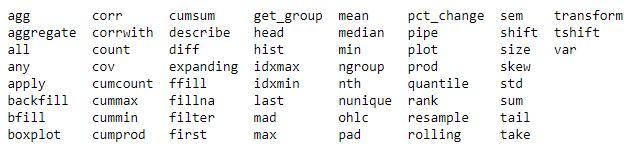Note, that in the code example above we also import IPython to print the list in columns. In the following examples we are going to use some of these methods. First, we can print out the groups by using the groups method to get a dictionary of groups:

`df_rank.groups`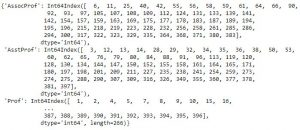We can also use the groupby method get_group to filter the grouped data. In the next code example we are going to select the Assistant Professor group (i.e., “AsstProf”).

```# Get group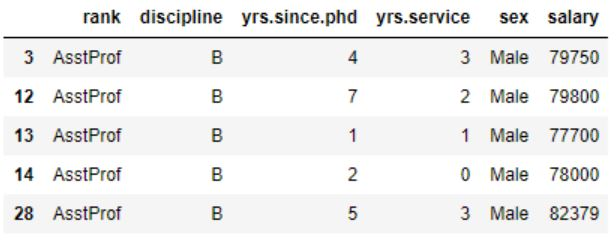### Pandas Groupby Count

If we want to find out how big each group is (e.g., how many observations in each group), we can use use .size() to count the number of rows in each group:

```df_rank.size()

# Output:
#
# rank
# AssocProf     64
# AsstProf      67
# Prof         266
# dtype: int64```

Additionally, we can also use Pandas groupby count method to count by group(s) and get the entire dataframe. If we don’t have any missing values the number should be the same for each column and group. Thus, this is a way we can explore the dataset and see if there are any missing values in any column.

`df_rank.count()`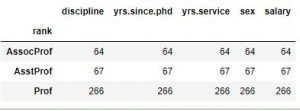That was how to use Pandas size to count the number of rows in each group. We will return to this, later, when we are grouping by multiple columns. Now we are going to In some cases we may want to find out the number of unique values in each group. This can be done using the groupby method nunique:

`df_rank.nunique()`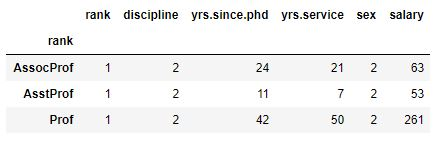As can be seen in the the last column (salary) there are 63 Associate Professors, 53 Assistant Proffessors, and 261 Professors in the dataset. In this example we have a complete dataset and we can see that some have the same salary (e.g., there are 261 unique values in the column salary for Professors). As we will see if we have missing values in the dataframe we would get a different result. In the next example we are using Pandas mask method together with NumPy’s random.random to insert missing values (i.e., np.NaN) in 10% of the dataframe:

```df_null = df.mask(np.random.random(df.shape) < .1)
df_null.isnull().sum().reset_index(name='N Missing Values')```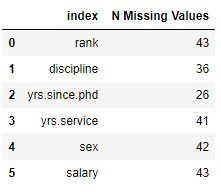Note, we used the reset_index method above to get the multi-level indexed grouped dataframe to become a single indexed. In the particular example, above, we used the parameter name to name the count column (“N Missing Values”). This parameter, however, can only be used on Pandas series objects and not dataframe objects.

That said, let’s return to the example; if we run the same code as above (counting unique values by group) we can see that it will not count missing values:

```df_null.groupby('rank').nunique()
```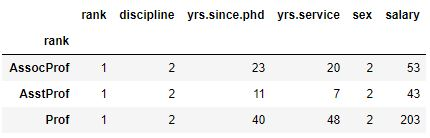That is, we don’t get the same numbers in the two tables because of the missing values. In the following examples we are going to work with Pandas groupby to calculate the mean, median, and standard deviation by one group.

### Pandas Groupby Mean

If we want to calculate the mean salary grouped by one column (rank, in this case) it’s simple. We just use Pandas mean method on the grouped dataframe:

`df_rank['salary'].mean().reset_index()`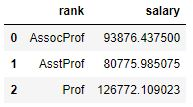Having a column named salary may not be useful. For instance, if someone else are going to see the table they may not know that it’s the mean salary for each group. Luckily, we can add the rename method to the above code to rename the columns of the grouped data:

```df_rank['salary'].mean().reset_index().rename(
columns={'rank':'Rank','salary' : 'Mean Salary'})
```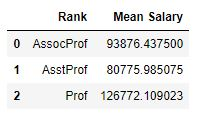### Median Score of a Group Using the groupby Method in Pandas

Now lets group by disciplne of the academic and find the median salary in the next Pandas groupby example

```df.groupby('rank')['salary'].median().reset_index().rename(
columns={'rank':'Rank','salary' : 'MedianSalary'})
```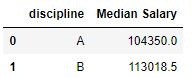### Aggregate Data by Group using Pandas Groupby

Most of the time we want to have our summary statistics in the same table. We can calculate the mean and median salary, by groups, using the agg method. In this next Pandas groupby example we are also adding the minimum and maximum salary by group (rank):

```df_rank['salary'].agg(['mean', 'median',
'std', 'min', 'max']).reset_index()```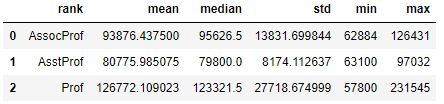A very neat thing with Pandas agg method is that we can write custom functions and pass them along. Let’s say that we wanted, instead of having one column for min salary and one column for max salary, to have a column with salary range:

```def salary_range(df):
mini = df.min()
maxi = df.max()
rang = '%s - %s' % (mini, maxi)

return rang

df_descriptive = df_rank['salary'].agg(['mean', 'median', 'std', salary_range]).reset_index()```

Here, however, the output will have the name of the methods/functions used. That is, we will have a column named ‘salary_range’ and we are going to rename this column:

```# Renaming Pandas Dataframe Columns
df_descriptive.rename(columns={'rank':'Rank', 'mean':'Mean', 'median':'Median',
'std':'Standard Deviation', 'salary_range':'Range'})```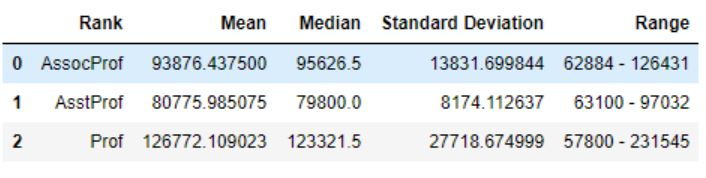Furthermore, it’s possible to use methods from other Python packages such as SciPy and NumPy. For instance, if we wanted to calculate the harmonic and geometric mean we can use SciPy:

```from scipy.stats.mstats import gmean, hmean

df_descriptive = df_rank['salary'].agg(['mean', 'median', hmean, gmean]).reset_index()
df_descriptive```

More about doing descriptive statistics using Pyton:

## Pandas Groupby Multiple Columns

In this section we are going to continue using Pandas groupby but grouping by many columns. In the first example we are going to group by two columns and the we will continue with grouping by two columns, ‘discipline’ and ‘rank’. To use Pandas groupby with multiple columns we add a list containing the column names. In the example below we also count the number of observations in each group:

```df_grp = df.groupby(['rank', 'discipline'])
df_grp.size().reset_index(name='count')```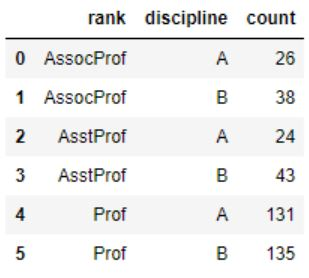Again, we can use the get_group method to select groups. However, in this case we have to input a tuple and select two groups:

```# Get two groups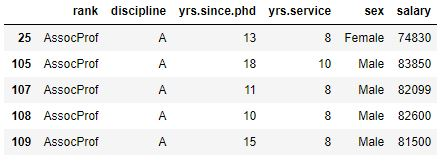## Pandas Groupby Count Multiple Groups

In the next groupby example we are going to calculate the number of observations in three groups (i.e., “n”). We have to start by grouping by “rank”, “discipline” and “sex” using groupby. As with the previous example (groupby one column) we use the method size to calculate the n and reset_index, with the parameter name=”n”, to get the series to a dataframe:

```df_3grps = df.groupby(['rank', 'discipline', 'sex'])
df_n_per_group = df_3grps.size().reset_index(name='n')```

Now we can continue and calculate the percentage of men and women in each rank and discipline. In this, and the next, Pandas groupby example we are going to use the apply method together with the lambda function.

```perc = df.groupby(['rank', 'discipline', 'sex'])['salary'].size()

# Give the percentage on the level of Rank:
percbyrank = perc.groupby(level=0).apply(lambda x: 100 * x / float(x.sum()))

print(percbyrank)
print('Total percentage in group AssocProf. ',
percbyrank.reset_index().query('rank == "AssocProf"')['salary'].sum())
```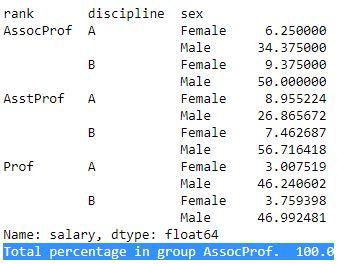Note, in the last line of code above we calculate the total of % for the group AssocProf and it’s 100, which is good. We are going to continue with calculating the percentage of men and women in each group (i.e., rank and discipline). In the next code we have to summarize the total (n=397). We can, for instance, see that there are more male professors regardless of discipline.

```n = perc.reset_index()['salary'].sum()
totalperc =  perc.groupby(level=0).apply(lambda x: 100 * x / N).reset_index(name='% of total n')
totalperc.reset_index()```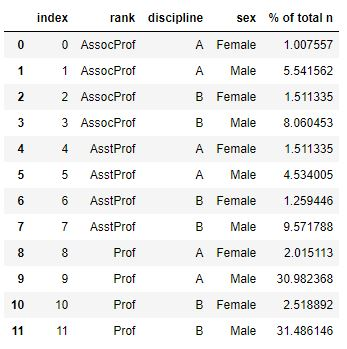### How to convert a Pandas groupby to Dataframe

When dealing with multiple groups and Pandas groupby we get a GroupByDataFrame object. Let’s use type to see what type a grouped object have:

`df_rn = df.groupby(['rank', 'discipline']).mean()`

Furthermore, if we use the index method we can see that it is MultiIndex:

`df_rn.index`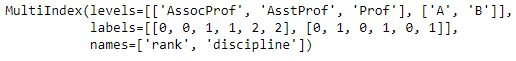It’s easy to convert the Pandas groupby to dataframe; we have actually already done it. In this example, however, we are going to calculate the mean values per the three groups. Furthermore, we are going to add a suffix to each column and use reset_index to get a dataframe.

```df_rn = df_rn.add_suffix('_Mean').reset_index()
type(df_rn)

# Output: pandas.core.frame.DataFrame```

## Pandas groupby agg with Multiple Groups

In this last section we are going use agg, again. We are not going into detail on how to use mean, median, and other methods to get summary statistics, however. This is because it’s basically the same as for grouping by n groups and it’s better to get all the summary statistics in one table.

That is, we are going to calculate mean, median, and standard deviation using the agg method. In this groupby example we are also adding the summary statistics (i.e., “mean”, “median”, and “std”) to each column. Otherwise we will get a multi-level indexed result like the image below: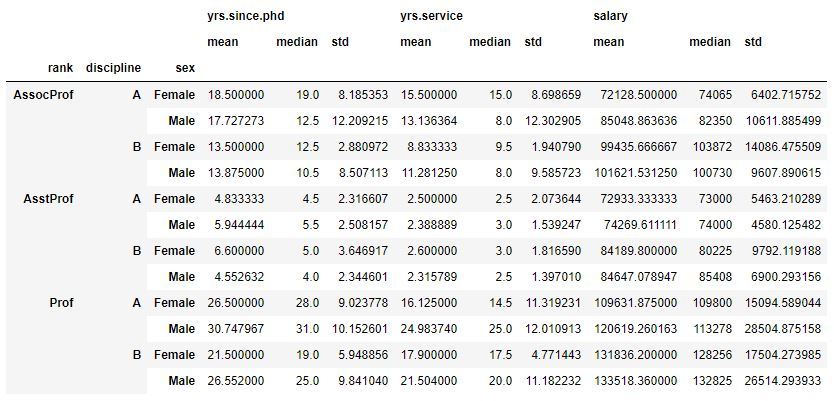If we use Pandas columns and the method ravel together with list comprehension we can add the suffixes to our column name and get another table. Note, in the example code below we only print the first 7 columns. In fact, with many columns it may be better to keep the result multi-level indexed.

```df_stats = df.groupby(['rank', 'discipline', 'sex']).agg(['mean', 'median', 'std'])
df_stats.columns = ["_".join(x) for x in df_stats.columns.ravel()]

df_stats.iloc[:,0:6].reset_index()```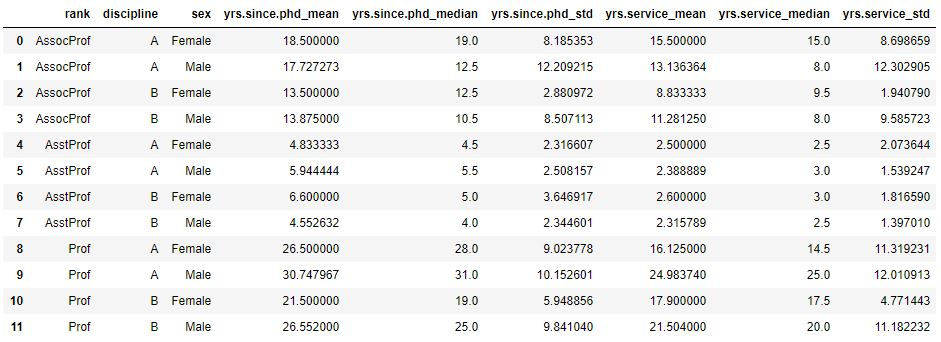Note, if we wanted an output as the first image we just remove the second line above (“df_stats.columns = …”). Additionally, as previous mentioned, we can also use custom functions, NumPy and SciPy methods when working with groupby agg. Just scroll back up and look at those examples, for grouping by one column, and apply them to the data grouped by multiple columns. More information of the different methods and objects used here can be found in the Pandas documentation.

## Conclusion:

In this Pandas groupby tutorial we have learned how to use Pandas groupby to:

• group one or many columns
• count observations using the methods count and size
• calculate simple summary statistics using:
• groupby mean, median, std
• groupby agg (aggregate)
• agg with our own function
• Calculate the percentage of observations in different groups

## Be First to Comment

This site uses Akismet to reduce spam. Learn how your comment data is processed.